### Create equations using the Equations pod

You create an equation by inserting an equation element and then entering the mathematical expres­sions for the equation. To insert an element, you can use the Element Catalog. You can also use a New Equation command from the Equations pop-up menu in the Equations pod.

note:   The term “math element” refers to part of an expression, such as an operator. It is not a structural element.

You can use any equation element for both inline and display equations. Some documents also have a paragraph element defined that provides formatting properties for the display equations.

The format rules for an equation element suggest a set of font sizes for the equation: Small, Medium, or Large. You can change to a different set of font sizes. This change is not considered a format rule override. If you remove format rule overrides in the document, the equation does not return to its original font size.

#### Create an inline equation using an element

1)Click in text where you want to insert the equation. If the text is in a rotated text frame, unro­tate the frame first by pressing Esc g 0 (zero).

2)Select an equation element in the Element Catalog and click Insert.

The first math item that you enter replaces the question mark prompt of the new equation object.

New equation object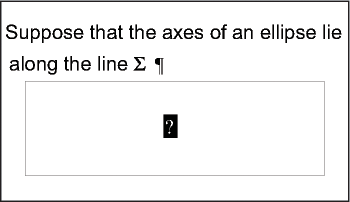3)Enter the equation by typing numerals and other items or by clicking items on the Equations pod.

4)Choose Shrink-Wrap Equation from the Equations pop-up menu in the pod.

Shrink-wrapped frame around an inline equation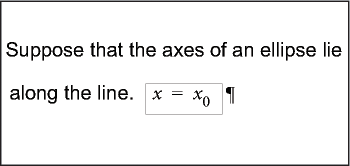5)If the equation seems too close to the text on either side, insert a space before or after the frame. FrameMaker treats a frame that contains an inline equation as a character and doesn’t provide extra space around it.

If an inline equation is too tall for its line, perhaps turn off fixed line spacing for that paragraph.

#### Create a display equation using an element

1)If your document has a paragraph element defined for formatting display equations, click where you want the equation paragraph. Select the paragraph element in the Element Catalog, and click Insert.

This element sometimes defines space above and below the equation, alignment in the text column, and an autonumbered caption.

2)Click in an empty paragraph element where you want to insert the equation.

3)Select an equation element in the Element Catalog, and click Insert.

New equation object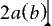4)Enter the equation.

5)Choose Shrink-Wrap Equation from the Equations pop-up menu.

Shrink-wrapped frame around a display equation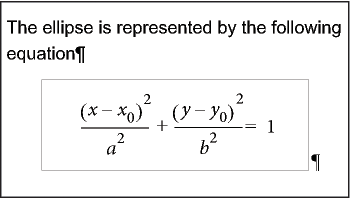6)If no equation element is available at the location that you want, perhaps you can use an invalid element. Do one of the following:

To use an element that is valid in another part of the document, insert the element in a valid location and then move it. You can also use the All Elements setting to make the element available everywhere and then insert the element where you want it.

To insert an invalid equation element with the default tag EQUATION, choose a New Equation command from the Equations pop-up menu in the Equations pod. The element has a default tag if no defined equation elements are available.

After inserting the element, talk to your developer about making the element valid at this loca­tion.

#### Create an equation in an anchored frame element

1)Select an anchored frame or a graphic object in the frame.

2)Choose a New Equation command from the Equations pop-up menu in the pod.

The first math item you enter replaces the question mark prompt of the new equation object. The equation does not appear in the document structure.

New equation object in a frame with graphic objects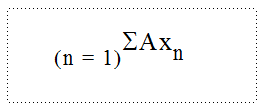3)Enter the equation.

September 30, 2016